Dining tables

In the dining room are tables with 4 chairs, 6 chairs, 8 chairs.
How many diners must be at least to be occupy all tables (chairs) and diners are more than 50?

Result

n =  72

Solution:Leave us a comment of example and its solution (i.e. if it is still somewhat unclear...):Be the first to comment!To solve this example are needed these knowledge from mathematics:

Do you want to calculate least common multiple two or more numbers?

Next similar examples:

1. BiketrialKamil was biketrial. Before hill he set the forward gear with 42 teeth and the back with 35 teeth. After how many exercises (rotation) of the front wheel both wheels reach the same position?
2. Matches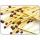George poured out of the box matches and composing them triangles and no match was left. Then he tries squares, hexagons and octagons and no match was left. How many matches must be at least in the box?
3. School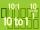Headteacher think whether the distribution of pupils in race in groups of 4,5,6,9 or 10. How many pupils must have at least school at possible options?
4. Apples 2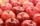How many minimum apples are in the cart, if possible is completely divided into packages of 6, 14 and 21 apples?
5. Dance ensemble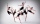The dance ensemble took the stage in pairs. During dancing, the dancers gradually formed groups of four, six and nine. How many dancers have an ensemble?
6. Cents no more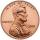Janko bought pencils for 35 cents each. Neither he nor the salesperson had small coins just a whole € 1 coin. At least how many pencils had to buy to pay for the whole euros?
7. Divisibility 2How many divisors has integer number 13?
8. LCM of two numberFind the smallest multiple of 63 and 147
9. 3 buses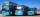At morning 5:00 am three buses started from one place. The first travel in five-minute intervals, the second at 10-minute intervals and the third at 25-minute intervals. At what hour will again be the three buses coming from the same place?
10. Three buses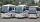Three public transport buses depart together from the bus station in the morning. The first bus was returning to the station after 18 minutes, the second after 12 minutes and a third after 24 minutes. How long will again together on the station? Result exp
11. LCMCommon multiple of three numbers is 3276. One number is in this number 63 times, second 7 times, third 9 times. What are the numbers?
12. Counting numberWhat is the smallest counting number divisible by 2,5,7,8 and 15?
13. Lcm of three numbersWhat is the Lcm of 120 15 and 5
14. Write decimalsWrite in the decimal system the short and advanced form of these numbers: a) four thousand seventy-nine b) five hundred and one thousand six hundred and ten c) nine million twenty-six
15. Prime factorsWrite 98 as product of prime factors
16. Two-digit numberI am a two-digit number less than 20. When I divided by three, then you get the rest 1 and when you divide me by four you get also rest 1. What number am I?
17. Primes 2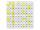Which prime numbers is number 2025 divisible?